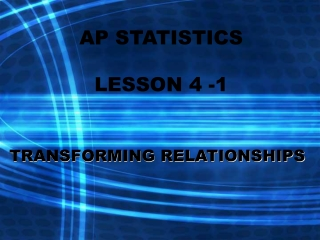DownloadDownload PresentationAP STATISTICS LESSON 4 -1

# AP STATISTICS LESSON 4 -1

Télécharger la présentation## AP STATISTICS LESSON 4 -1

- - - - - - - - - - - - - - - - - - - - - - - - - - - E N D - - - - - - - - - - - - - - - - - - - - - - - - - - -
##### Presentation Transcript

1. AP STATISTICSLESSON 4 -1 TRANSFORMING RELATIONSHIPS

2. ESSENTIAL QUESTION: How can curves be transformed into straight lines? Objectives: • To use inverse functions to transform data into linear equations. • To use the transformed data to create mathematical models that will make predictions using data.

3. Linearvs. Exponential growth • Linear growth increases by a fixed amount in each equal time period. • Exponential growth increases by a fixed percentage of the previous total.

4. Algebraic Properties of Logarithms logb x = y if and only by = x The rules for logarithms are: • log(AB) = log A + log B • log(A/B) = logA – log B 3. logXp = plog X